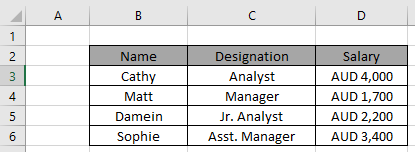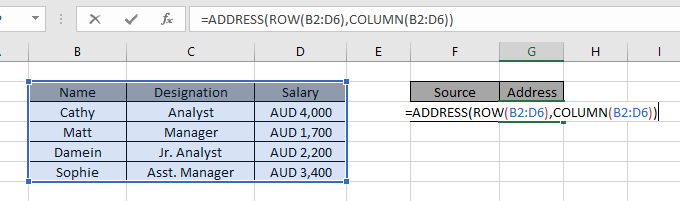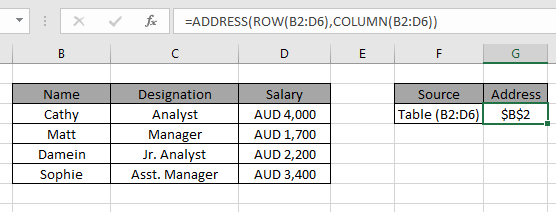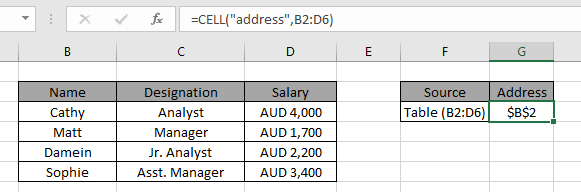# Find the Address of first cell in range in Excel

In this article, we will learn how to Find the Address of first cell in range in Excel.
At Instance, we need to find the first cell address as value for the data table. For DATA table we have a range of value values as array which is given as input to the formula and hence the formula returns the first cell value.
For this article we will be needing the use the following functions:

Below are some information about the above stated functions we will use.

The ADDRESS function simply returns the address of a cell by using row number and column number.

Syntax:

=ADDRESS ( row_num , col_num , [abs_num] )

Row_num: The Row number

Col_num: The Column number

[abs_num]: [Optional] number, possible inputs, 1, 2, 3, or 4. If omitted or 1, returned address will be absolute eg \$A\$1

Now we will make a formula out of these functions. Here we will given a data table. We need to find the first cell address for the data table

Use the formula:

= ADDRESS ( ROW ( table_array ) , COLUMN ( table_array ) )

Variables:
table_array: data table given as array

Let's test this formula via running it on an example

Here we have a data table and we need to find the first cell address for the same data table using the formula.Formula:

= ADDRESS ( ROW ( B2:D6 ), COLUMN ( B2:D6 ) )

B2:D6 : table array

Explanation

• COLUMN function returns the first column value from the table array.
• ROW function returns the first row number from the table array.
• ADDRESS function takes the row_num & column_value as arguments and returns its absolute reference.Here the array to the function is given as cell_reference. Press Enter to get the result.As you can see in the formula works fine as it returns the first cell value for the data table.

Alternate method using the CELL function:

CELL function is a built-in Excel Info function. Cell function in Excel stores all the data of a referenced cell and returns the info_type of the cell.

Formula:

= CELL ( "address" , [array_reference] )

Variables:
[array_reference] : table array given as reference.As you can see from the above formula the you can get the first cell value as reference.

Hope this article about how to Find the Address of first cell in range in Excel is explanatory. Find more articles on lookup last value via functions here. Please share your query below in the comment box. We will assist you.

Related Articles

How to use the MATCH function : Return the position of the value in a list.

How to use the ADDRESS function : Returns the absolute or relative reference of the cell address.

Finding the Last Day of a Given Month : Returns the last day of a given month.

How to Get Last Value In Column : Find the last value in a column or list.

Difference with the last non blank cell : Returns the SUM of values between given dates or period in excel.

Popular Articles

50 Excel Shortcut to Increase Your Productivity

Edit a dropdown list

Absolute reference in Excel

If with conditional formatting

If with wildcards

Vlookup by date

Terms and Conditions of use

The applications/code on this site are distributed as is and without warranties or liability. In no event shall the owner of the copyrights, or the authors of the applications/code be liable for any loss of profit, any problems or any damage resulting from the use or evaluation of the applications/code.